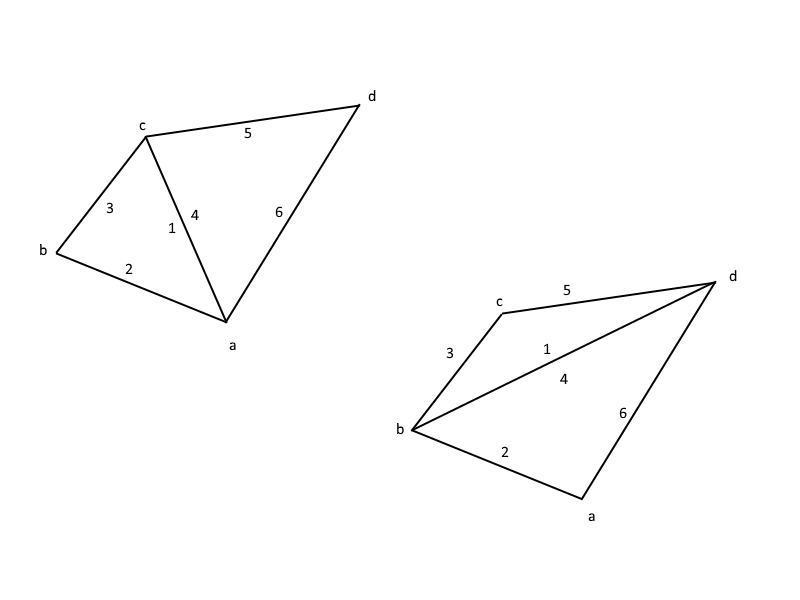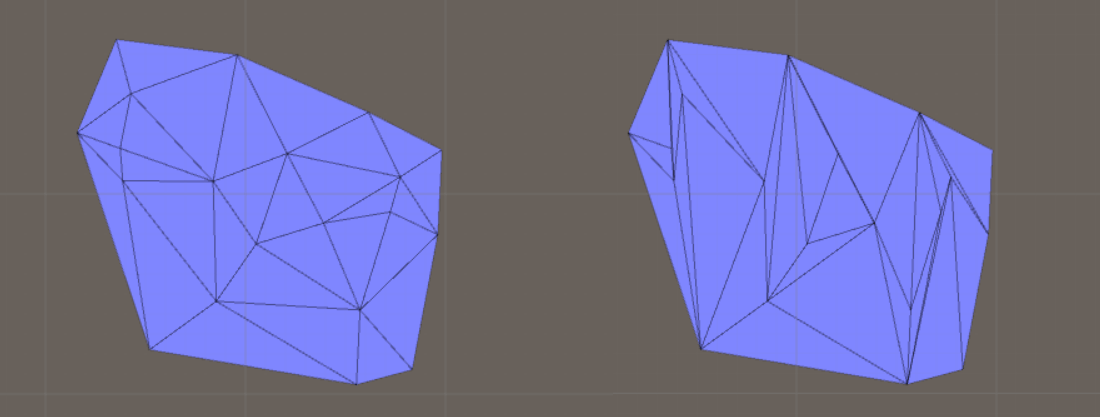# Use math to solve problems in Unity with C#

## 11. Delaunay Triangulation

Here you will learn how to triangulate points with the Delaunay Triangulation algorithm. Remember to use the data structures from the first page, such as Vertex and Triangle, and everything should be in x-z-space (Create a new Vertex object with a Vector3 as its position and where y = 0).

What we saw in the last section of this tutorial, Triangulation of random points, was that it's easy to triangulate random points. But the resulting triangles were ugly, which doesnt matter in most cases, but what if you want more good-looking triangles that have the same size, then you have to use the Delaunay Triangulation algorithm.

As usual there are more than one way to achieve a Delaunay Triangulation, and we are here going to use the simplest one. We are first going to use one of the algortihms from Triangulation of random points, so we will have some triangles that we need to change by flipping edges. To make it easier to flip edges, we have to change data structure from triangles to half-edge with this method:

```//From triangle where each triangle has one vertex to half edge
public static List<HalfEdge> TransformFromTriangleToHalfEdge(List<Triangle> triangles)
{
//Make sure the triangles have the same orientation
MeshOperations.OrientTrianglesClockwise(triangles);

//First create a list with all possible half-edges
List<HalfEdge> halfEdges = new List<HalfEdge>(triangles.Count * 3);

for (int i = 0; i < triangles.Count; i++)
{
Triangle t = triangles[i];

HalfEdge he1 = new HalfEdge(t.v1);
HalfEdge he2 = new HalfEdge(t.v2);
HalfEdge he3 = new HalfEdge(t.v3);

he1.nextEdge = he2;
he2.nextEdge = he3;
he3.nextEdge = he1;

he1.prevEdge = he3;
he2.prevEdge = he1;
he3.prevEdge = he2;

//The vertex needs to know of an edge going from it
he1.v.halfEdge = he2;
he2.v.halfEdge = he3;
he3.v.halfEdge = he1;

//The face the half-edge is connected to
t.halfEdge = he1;

he1.t = t;
he2.t = t;
he3.t = t;

//Add the half-edges to the list
}

//Find the half-edges going in the opposite direction
for (int i = 0; i < halfEdges.Count; i++)
{
HalfEdge he = halfEdges[i];

Vertex goingToVertex = he.v;
Vertex goingFromVertex = he.prevEdge.v;

for (int j = 0; j < halfEdges.Count; j++)
{
//Dont compare with itself
if (i == j)
{
continue;
}

HalfEdge heOpposite = halfEdges[j];

//Is this edge going between the vertices in the opposite direction
if (goingFromVertex.position == heOpposite.v.position && goingToVertex.position == heOpposite.prevEdge.v.position)
{
he.oppositeEdge = heOpposite;

break;
}
}
}

return halfEdges;
}
```

...which requires a method to make sure all triangles have the same orientation:

```//Orient triangles so they have the correct orientation
public static void OrientTrianglesClockwise(List<Triangle> triangles)
{
for (int i = 0; i < triangles.Count; i++)
{
Triangle tri = triangles[i];

Vector2 v1 = new Vector2(tri.v1.position.x, tri.v1.position.z);
Vector2 v2 = new Vector2(tri.v2.position.x, tri.v2.position.z);
Vector2 v3 = new Vector2(tri.v3.position.x, tri.v3.position.z);

if (!Geometry.IsTriangleOrientedClockwise(v1, v2, v3))
{
tri.ChangeOrientation();
}
}
}
```

...which requires a method to check which orientation a triangle has:

```//Is a triangle in 2d space oriented clockwise or counter-clockwise
//https://math.stackexchange.com/questions/1324179/how-to-tell-if-3-connected-points-are-connected-clockwise-or-counter-clockwise
//https://en.wikipedia.org/wiki/Curve_orientation
public static bool IsTriangleOrientedClockwise(Vector2 p1, Vector2 p2, Vector2 p3)
{
bool isClockWise = true;

float determinant = p1.x * p2.y + p3.x * p1.y + p2.x * p3.y - p1.x * p3.y - p3.x * p2.y - p2.x * p1.y;

if (determinant > 0f)
{
isClockWise = false;
}

return isClockWise;
}
```

We will now loop through all half-edges and check if we need to flip the edge to make a better triangle. To check if we need to flip and edge we are going to use the circle test. Each edge shares two triangles. Then you draw a circle where the corners of one triangles are on the boundary of the circle. If the corner of the opposite triangle is within this circle, then we should flip the edge. So add this method:

```//Is a point d inside, outside or on the same circle as a, b, c
//Returns positive if inside, negative if outside, and 0 if on the circle
public static float IsPointInsideOutsideOrOnCircle(Vector2 aVec, Vector2 bVec, Vector2 cVec, Vector2 dVec)
{
//This first part will simplify how we calculate the determinant
float a = aVec.x - dVec.x;
float d = bVec.x - dVec.x;
float g = cVec.x - dVec.x;

float b = aVec.y - dVec.y;
float e = bVec.y - dVec.y;
float h = cVec.y - dVec.y;

float c = a * a + b * b;
float f = d * d + e * e;
float i = g * g + h * h;

float determinant = (a * e * i) + (b * f * g) + (c * d * h) - (g * e * c) - (h * f * a) - (i * d * b);

return determinant;
}
```

But we cant always flip an edge, we also have to make sure the area formed by the two triangles that share an edge is convex. Otherwise the flipped edge will end up outside of the area. A fast method to determine this is:

```//Is a quadrilateral convex? Assume no 3 points are colinear and the shape doesnt look like an hourglass
public static bool IsQuadrilateralConvex(Vector2 a, Vector2 b, Vector2 c, Vector2 d)
{
bool isConvex = false;

bool abc = Geometry.IsTriangleOrientedClockwise(a, b, c);
bool abd = Geometry.IsTriangleOrientedClockwise(a, b, d);
bool bcd = Geometry.IsTriangleOrientedClockwise(b, c, d);
bool cad = Geometry.IsTriangleOrientedClockwise(c, a, d);

if (abc && abd && bcd & !cad)
{
isConvex = true;
}
else if (abc && abd && !bcd & cad)
{
isConvex = true;
}
else if (abc && !abd && bcd & cad)
{
isConvex = true;
}
//The opposite sign, which makes everything inverted
else if (!abc && !abd && !bcd & cad)
{
isConvex = true;
}
else if (!abc && !abd && bcd & !cad)
{
isConvex = true;
}
else if (!abc && abd && !bcd & !cad)
{
isConvex = true;
}

return isConvex;
}
```

Now we can finally take a look at the main Delaunay Triangle method:

```//Alternative 1. Triangulate with some algorithm - then flip edges until we have a delaunay triangulation
public static List<Triangle> TriangulateByFlippingEdges(List<Vector3> sites)
{
//Step 1. Triangulate the points with some algorithm
//Vector3 to vertex
List<Vertex> vertices = new List<Vertex>();

for (int i = 0; i < sites.Count; i++)
{
}

//Triangulate the convex hull of the sites
List<Triangle> triangles = TriangulatePoints.IncrementalTriangulation(vertices);
//List triangles = TriangulatePoints.TriangleSplitting(vertices);

//Step 2. Change the structure from triangle to half-edge to make it faster to flip edges
List<HalfEdge> halfEdges = TransformRepresentation.TransformFromTriangleToHalfEdge(triangles);

//Step 3. Flip edges until we have a delaunay triangulation
int safety = 0;

int flippedEdges = 0;

while (true)
{
safety += 1;

if (safety > 100000)
{
Debug.Log("Stuck in endless loop");

break;
}

bool hasFlippedEdge = false;

//Search through all edges to see if we can flip an edge
for (int i = 0; i < halfEdges.Count; i++)
{
HalfEdge thisEdge = halfEdges[i];

//Is this edge sharing an edge, otherwise its a border, and then we cant flip the edge
if (thisEdge.oppositeEdge == null)
{
continue;
}

//The vertices belonging to the two triangles, c-a are the edge vertices, b belongs to this triangle
Vertex a = thisEdge.v;
Vertex b = thisEdge.nextEdge.v;
Vertex c = thisEdge.prevEdge.v;
Vertex d = thisEdge.oppositeEdge.nextEdge.v;

Vector2 aPos = a.GetPos2D_XZ();
Vector2 bPos = b.GetPos2D_XZ();
Vector2 cPos = c.GetPos2D_XZ();
Vector2 dPos = d.GetPos2D_XZ();

//Use the circle test to test if we need to flip this edge
if (Geometry.IsPointInsideOutsideOrOnCircle(aPos, bPos, cPos, dPos) < 0f)
{
//Otherwise the edge cant be flipped
{
//If the new triangle after a flip is not better, then dont flip
//This will also stop the algoritm from ending up in an endless loop
if (Geometry.IsPointInsideOutsideOrOnCircle(bPos, cPos, dPos, aPos) < 0f)
{
continue;
}

//Flip the edge
flippedEdges += 1;

hasFlippedEdge = true;

FlipEdge(thisEdge);
}
}
}

//We have searched through all edges and havent found an edge to flip, so we have a Delaunay triangulation!
if (!hasFlippedEdge)
{
//Debug.Log("Found a delaunay triangulation");

break;
}
}

//Debug.Log("Flipped edges: " + flippedEdges);

//Dont have to convert from half edge to triangle because the algorithm will modify the objects, which belongs to the
//original triangles, so the triangles have the data we need

return triangles;
}
```

...which requires a method that flips an edge:

```//Flip an edge
private static void FlipEdge(HalfEdge one)
{
//The data we need
//This edge's triangle
HalfEdge two = one.nextEdge;
HalfEdge three = one.prevEdge;
//The opposite edge's triangle
HalfEdge four = one.oppositeEdge;
HalfEdge five = one.oppositeEdge.nextEdge;
HalfEdge six = one.oppositeEdge.prevEdge;
//The vertices
Vertex a = one.v;
Vertex b = one.nextEdge.v;
Vertex c = one.prevEdge.v;
Vertex d = one.oppositeEdge.nextEdge.v;

//Flip

//Change vertex
a.halfEdge = one.nextEdge;
c.halfEdge = one.oppositeEdge.nextEdge;

//Change half-edge
//Half-edge - half-edge connections
one.nextEdge = three;
one.prevEdge = five;

two.nextEdge = four;
two.prevEdge = six;

three.nextEdge = five;
three.prevEdge = one;

four.nextEdge = six;
four.prevEdge = two;

five.nextEdge = one;
five.prevEdge = three;

six.nextEdge = two;
six.prevEdge = four;

//Half-edge - vertex connection
one.v = b;
two.v = b;
three.v = c;
four.v = d;
five.v = d;
six.v = a;

//Half-edge - triangle connection
Triangle t1 = one.t;
Triangle t2 = four.t;

one.t = t1;
three.t = t1;
five.t = t1;

two.t = t2;
four.t = t2;
six.t = t2;

//Opposite-edges are not changing!

//Triangle connection
t1.v1 = b;
t1.v2 = c;
t1.v3 = d;

t2.v1 = b;
t2.v2 = d;
t2.v3 = a;

t1.halfEdge = three;
t2.halfEdge = four;
}
```

...which requires an image to describe what's going on:...and if everything works as it should, you will end up with the figure to the left (the figure to the right shows the same triangulation but before we began flipping triangles):